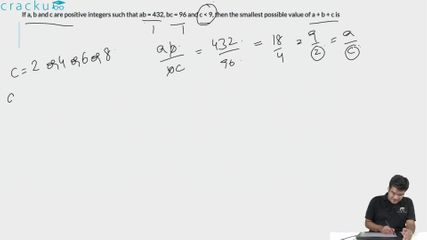Question 72

# If a, b and c are positive integers such that ab = 432, bc = 96 and c < 9, then the smallest possible value of a + b + c is

Solution

Since $$c<9$$, we can have the following viable combinations for $$b\times\ c\ =96$$ (given our objective is to minimize the sum):

$$48\times\ 2$$ ; $$32\times3$$ ; $$24\times\ 4$$ ; $$16\times6$$ ; $$12\times8$$

Similarly, we can factorize $$a\times\ b\ = 432$$ into its factors. On close observation, we notice that $$18\times24\ and\ 24\ \times\ 4\$$ corresponding to $$a\times b\ and\ b\times\ c\$$ respectively together render us with the least value of the sum of $$a+b\ +\ c\ \ =\ 18+24+4\ =46$$

Hence, Option D is the correct answer.

### View Video Solution